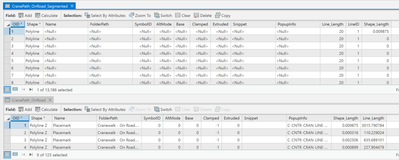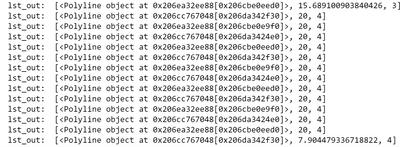# Help in segmenting a polyline layer into equally length segments

1075
15
03-18-2021 02:35 PMNew Contributor II

Hello, I have a script that my intentions are for it to segment polylines into equal intervals with the last polyline as a remainder. Initially, I attempted to conduct this through GeneratePointsAlongLines at equal distance and then SplitLinesAtPoint functions but noticed that this produced segmented lines that had lengths that were inconsistent with the required length. Furthermore, I'm the only person at my company that has an Advanced Pro license and I would like to make this tool work for others.

My script is able to successfully iterate through the source polylines layer and add information to an output layer using cursors and segmentAlongLine method. Though the attributes table has the correct number of rows, the feature layer still only shows the original polyline when selected. I believe what is happening is that the segmented polyline doesn't actually exist. Any ideas on what I'm doing wrong? Thank You!
Below is my script:import arcpy
arcpy.env.overwriteOutput = True
arcpy.env.workspace = r"C:\Users\roder\OneDrive\Documents\ArcGIS\Projects\PythonScripting\PythonScripting.gdb" # Change to getParameter
spatial_ref = arcpy.Describe(polylines).spatialReference
output = "CranePath_OnRoad_Segmented" #Change to getParameter
interval_dist = 20 # feet, Change to get Parameter
#distance_interval_unit = getParameter
arcpy.management.CalculateGeometryAttributes(polylines, "Line_Length LENGTH_GEODESIC", "FEET_US")
mem_polyline = arcpy.CreateFeatureclass_management("in_memory", "mem_polyline", "POLYLINE", polylines, "DISABLED", "DISABLED", spatial_ref)
in_flds = ["OID@", "SHAPE@", "Line_Length"]
out_flds = ["SHAPE@", "Line_Length", "LineID"]
cnt_in = 0
cnt_out = 0
with arcpy.da.InsertCursor(mem_polyline,out_flds) as curs_out:
with arcpy.da.SearchCursor(polylines,in_flds) as curs_in:
for row_in in curs_in:
cnt_in += 1
polyline_in = row_in
from_dist = 0
lineID = row_in
max_dist = float(row_in)
if cnt_in % 100 == 0:
print("Processing line: {}, generated {} segments".format(cnt_in, cnt_out))

while from_dist <= max_dist:
cnt_out += 1
to_dist = from_dist + interval_dist
remainder_dist = max_dist - from_dist
segment = polyline_in.segmentAlongLine(from_dist,to_dist,False)
lst_out = list((segment,interval_dist,lineID))
curs_out.insertRow(lst_out)
from_dist += interval_dist
else:
cnt_out += 1
segment = polyline_in.segmentAlongLine(from_dist-interval_dist,max_dist,False)
lst_out = list((segment,remainder_dist,lineID))
curs_out.insertRow(lst_out)

mem_polyline_fl = arcpy.MakeFeatureLayer_management(mem_polyline, "Polylines_memory")
arcpy.CopyFeatures_management(mem_polyline_fl, output)
arcpy.Delete_management(mem_polyline)
arcpy.Delete_management(mem_polyline_fl)

Tags (2)
15 RepliesbyMVP Esteemed Contributor

Code formatting ... the Community Version - Esri Community

... sort of retired...New Contributor II
``````import arcpy
arcpy.env.overwriteOutput = True
arcpy.env.workspace = r"C:\Users\roder\OneDrive\Documents\ArcGIS\Projects\PythonScripting\PythonScripting.gdb" # Change to getParameter
spatial_ref = arcpy.Describe(polylines).spatialReference
output = "CranePath_OnRoad_Segmented" #Change to getParameter
interval_dist = 20 # feet, Change to get Parameter
#distance_interval_unit = getParameter
arcpy.management.CalculateGeometryAttributes(polylines, "Line_Length LENGTH_GEODESIC", "FEET_US")
mem_polyline = arcpy.CreateFeatureclass_management("in_memory", "mem_polyline", "POLYLINE", polylines, "DISABLED", "DISABLED", spatial_ref)
in_flds = ["OID@", "SHAPE@", "Line_Length"]
out_flds = ["SHAPE@", "Line_Length", "LineID"]
cnt_in = 0
cnt_out = 0
with arcpy.da.InsertCursor(mem_polyline,out_flds) as curs_out:
with arcpy.da.SearchCursor(polylines,in_flds) as curs_in:
for row_in in curs_in:
cnt_in += 1
polyline_in = row_in
from_dist = 0
lineID = row_in
max_dist = float(row_in)
if cnt_in % 100 == 0:
print("Processing line: {}, generated {} segments".format(cnt_in, cnt_out))

while from_dist <= max_dist:
cnt_out += 1
to_dist = from_dist + interval_dist
remainder_dist = max_dist - from_dist
segment = polyline_in.segmentAlongLine(from_dist,to_dist,False)
lst_out = list((segment,interval_dist,lineID))
curs_out.insertRow(lst_out)
from_dist += interval_dist
else:
cnt_out += 1
segment = polyline_in.segmentAlongLine(from_dist-interval_dist,max_dist,False)
lst_out = list((segment,remainder_dist,lineID))
curs_out.insertRow(lst_out)

mem_polyline_fl = arcpy.MakeFeatureLayer_management(mem_polyline, "Polylines_memory")
arcpy.CopyFeatures_management(mem_polyline_fl, output)
arcpy.Delete_management(mem_polyline)
arcpy.Delete_management(mem_polyline_fl)``````byMVP Regular Contributor

Have to ask... is that layer turned on as selectable in the TOC?  Have you stepped through it with a debugger and checked out your values you are getting before being inserted?

If you are also turning this script into a tool, you can use a little conditional to assign a default value while running it as a standalone script so you don't have to keep converting polylines from polylines = getParamter() and polylines = 'CranePath_OnRoad'

``````polylines = GetParameter(1)
if polylines == '#' or not polylines:
polylines = "CranePath_OnRoad" # defaults to the set variable for standalone script operation since getParameter returns a hash when it is not set.``````New Contributor II

Hello Jeff,

Yes the source layer is selectable in the TOC. I reran the script with a fresh source to make sure. Interesting enough after the script is the run the source layer is removed from the map visually but still exists under the TOC and Catalog panes with appropriate check boxes turned on. Also thankyou for the tip and will introduce it once I get this script working correctly.

RoderickNew Contributor II

the only debugging that I've done is set a print statement for the 'lst_out' list. Here is a snippet of it:byMVP Regular Contributor

``arcpy.management.CalculateGeometryAttributes(polylines, "Line_Length LENGTH_GEODESIC", "FEET_US")``

The docs show it takes a list for the geometry_property parameter like:

``arcpy.CalculateGeometryAttributes_management(polylines, ["Line_Length", "LENGTH_GEODESIC"], "FEET_US")``

Another thing that might be an issue is not converting the values to float for the segmentAlongLine method.

``segment = polyline_in.segmentAlongLine(float(from_dist), float(to_dist), False)``New Contributor II

Hello Jeff,

I attempted to use the calculate geometry as listed in the API Reference document but couldn't get it to run and got the same error as when trying to run your code. However, what I did was run the calculate Geometry from GUI window and looked at the history detail. From there I was given the code I used, but I agree I wish there weren't two formats for the same function.

``````---------------------------------------------------------------------------
ExecuteError                              Traceback (most recent call last)
In  :
Line 10:    arcpy.CalculateGeometryAttributes_management(polylines, ["Line_Length", "LENGTH_GEODESIC"], "FEET_US")

File C:\Program Files\ArcGIS\Pro\Resources\ArcPy\arcpy\management.py, in CalculateGeometryAttributes:
Line 3820:  raise e

File C:\Program Files\ArcGIS\Pro\Resources\ArcPy\arcpy\management.py, in CalculateGeometryAttributes:
Line 3817:  retval = convertArcObjectToPythonObject(gp.CalculateGeometryAttributes_management(*gp_fixargs((in_features, geometry_property, length_unit, area_unit, coordinate_system, coordinate_format), True)))

File C:\Program Files\ArcGIS\Pro\Resources\ArcPy\arcpy\geoprocessing\_base.py, in <lambda>:
Line 511:   return lambda *args: val(*gp_fixargs(args, True))

ExecuteError: Traceback (most recent call last):
File "c:\program files\arcgis\pro\Resources\ArcToolbox\scripts\calculategeometry.py", line 269, in <module>
CalculateGeometry(fc, properties, lUnit, aUnit, cs, cformat)
File "c:\program files\arcgis\pro\Resources\ArcToolbox\scripts\calculategeometry.py", line 101, in CalculateGeometry
for row in ucur:
RuntimeError: Cannot find field 'LENGTH_GEODESIC'

Failed to execute (CalculateGeometryAttributes).``````byMVP Regular Contributor

That error says its not finding the 'Length Geodesic' field, which can be added by using the AddGeometryAttributes method

``````arcpy.AddGeometryAttributes_management(in_features, properties, length_unit,
area_unit,
coordinate_system)``````New Contributor II

Hey Jeff, I do really appreciate the help on this. I attempted to add float into the segment function but resulted in same output.